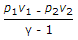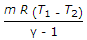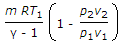# Mechanical Engineering - Thermodynamics

### Exercise :: Thermodynamics - Section 4

6.

The principal constituents of a fuel are

 A. carbon and hydrogen B. oxygen and hydrogen C. sulphur and oxygen D. sulphur and hydrogen

Explanation:

No answer description available for this question. Let us discuss.

7.

In an extensive property of a thermodynamic system

 A. extensive heat is transferred B. extensive work is done C. extensive energy is utilised D. none of these

Explanation:

No answer description available for this question. Let us discuss.

8.

When a gas is heated at constant pressure

 A. its temperature will increase B. its volume will increase C. both temperature and volume will increase D. neither temperature not volume will increase

Explanation:

No answer description available for this question. Let us discuss.

9.

By decreasing the highest temperature in the Carnot cycle, its efficiency is increased.

 A. True B. False

Explanation:

No answer description available for this question. Let us discuss.

10.

Workdone during adiabatic expansion is given by (where p1 v1, T1 = Pressure, volume and temperature for the initial condition of gas, p2, v2, T2 = Corresponding values for the final condition of gas, R = Gas constant, and γ = Ratio of specific heats)

 A.B.C.D. all of these